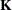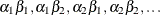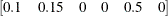# Shared Concepts and Topics

### Positional and Nonpositional Syntax for Coefficients in Linear Functions

When you define custom linear hypotheses with the ESTIMATE statement, the procedure sets up anvector or matrix that conforms to the model effect solutions. (Note that the following remarks also apply to the LSMESTIMATE statement, where you specify coefficients of the matrixwhich is then converted into a coefficient matrix that conforms to the model effects solutions.)

There are two methods for specifying the entries in a coefficient matrix (hereafter simply referred to as thematrix); they are called the positional and nonpositional methods. In the positional form, which is the traditional method, you provide a list of values that occupy the elements of thematrix that is associated with the effect in question in the order in which the values are listed. For traditional model effects that consist of continuous and classification variables, the positional syntax is simpler in some cases (main effects) and more cumbersome in others (interactions). When you work with effects that are constructed through the EFFECT statement, the nonpositional syntax is essential.

For example, consider the following two-way model with interactions where factors A and B have three and two levels, respectively:

proc logistic;
class a b;
model y = a b a*b;
run;


To test the difference of the B levels at the second level of A with an ESTIMATE statement (a slice), you need to assign coefficients 1 and –1 to the levels of B and to the levels of the interaction where A is at the second level. Two examples of equivalent ESTIMATE statements that use positional and nonpositional syntax are as follows:

estimate 'B at A2' b 1 -1 a*b 0  0  1 -1      ;
estimate 'B at A2' b 1 -1 a*b [1 2 1] [-1 2 2];


Because A precedes B in the CLASS statement, the levels of the interaction are formed as. If B precedes A in the CLASS statement, you need to modify the coefficients accordingly:

proc logistic;
class b a;
model y = a b a*b;
estimate 'B at A2' b 1 -1 a*b 0  1  0  0 -1     ;
estimate 'B at A2' b 1 -1 a*b [1  1 2] [-1  2 2];
estimate 'B at A2' b 1 -1 a*b [1, 1 2] [-1, 2 2];
run;


You can optionally separate thevalue entry from the level indicators with a comma, as in the last ESTIMATE statement.

The general syntax for defining coefficients with the nonpositional syntax is as follows:

effect-name [multiplier <,> level-values] …<[multiplier <,> level-values]>

The first entry in square brackets is the multiplier that is applied to the elements offor the effect after the level-values have been resolved and any necessary action that formshas been taken.

The level-values are organized in a specific form:

• The number of entries should equal the number of terms that are needed to construct the effect. For effects that do not contain any constructed effects, this number is simply the number of terms in the name of the effect.

• Values of continuous variables that are needed for the construction of thematrix precede the level indicators of CLASS variables.

• If the effect involves constructed effects, then you need to provide as many continuous and classification variables as are needed for the effect formation. For example, if a collection effect is defined as

class c;
effect v = collection(x1 x2 c);


then a proper nonpositional syntax would be

v [0.5,  0.2 0.3 3]

• If an effect contains both regular terms (old-style effects) and constructed effects, then the order of the coefficients is as follows: continuous values for old-style effects, class levels for classification variables in old-style effects, continuous values for constructed effects, and finally class levels that are needed for constructed effects. Assume that C has four levels so that effect v contributes six elements to thematrix. When the procedure resolves this syntax, the values 0.2 and 0.3 are assigned to the positions for x1 and x2 and a 1 is associated with the third level of C. The resulting vector is then multiplied by 0.5 to produceNote that you enter the levels of the classification variables in the square brackets, not their formatted values. The ordering of the levels of classification variables can be gleaned from the "Class Level Information" table.

To specify values for continuous variables, simply give their value as one of the terms in the effect. The nonpositional syntax in the following ESTIMATE statement is read as "1 times the value 0.4 in the column that is associated with level 2 of A"

proc phreg;
class a / param=glm;
model y = a a*x / s;
lsmeans a / e at x=0.4;
estimate 'A2 at x=0.4' intercept 1 a 0 1 a*x [1,0.4 2] / e;
run;


Because the value before the comma serves as a multiplier, the same estimable function could also be constructed with the following statements:

estimate 'A2 at x=0.4' intercept 1 a 0 1 a*x [ 4,  0.1 2];
estimate 'A2 at x=0.4' intercept 1 a 0 1 a*x [ 2,  0.2 2];
estimate 'A2 at x=0.4' intercept 1 a 0 1 a*x [-1, -0.4 2];


Note that continuous variables that are needed to construct an effect are always listed before any CLASS variables.

When you work with constructed effects, the nonpositional syntax works in the same way. For example, the following model contains a classification effect and a B-spline. The first two ESTIMATE statements produce predicted values for level 1 of C when the continuous variable x takes on the values 20 and 10, respectively.

proc orthoreg;
class c;
effect spl = spline(x / knotmethod=equal(5));
model y = c spl;
estimate 'C = 1 @ x=20' intercept 1 c 1 spl [1,20],
'C = 1 @ x=10' intercept 1 c 1 spl [1,10];
estimate 'Difference'   spl [1,20] [-1,10];
run;


In this example, the ORTHOREG procedure computes the spline coefficients for the first ESTIMATE statement based on x = 20, and similarly in the second statement for x = 10. The third ESTIMATE statement computes the difference of the predicted values. Because the spline effect does not interact with the classification variable, this difference does not depend on the level of C. If such an interaction is present, you can estimate the difference in predicted values for a given level of C by using the nonpositional syntax. Because the effect C*spl contains both old-style terms (C) and a constructed effect, you specify the values for the old-style terms before assigning values to constructed effects.

proc orthoreg;
class c;
effect spl = spline(x / knotmethod=equal(5));
model y = spl*c;
estimate 'C2 = 1, x=20' intercept 1 c*spl [1,1 20];
estimate 'C2 = 2, x=20' intercept 1 c*spl [1,2 20];
estimate 'C diff at x=20' c*spl [1,1 20] [-1,2 20];
run;


It is recommended that you add the E option to the ESTIMATE or LSMESTIMATE statement to verify that thematrix is formed according to your expectations.

In any row of an ESTIMATE statement you can choose positional and nonpositional syntax separately for each effect. However, you cannot mix the two forms of syntax for coefficients of a single effect. For example, the following statement is not proper because both forms of syntax are used for the interaction effect:

estimate 'A1B1 - A1B2' b 1 -1  a*b 0 1  [-1, 1 2];